# u3a - Maths Challenge - Week 6

## Maths Challenge - Week 6

• Published : 14 May 2020
• Views: 3093

Welcome to week 6 of our weekly maths challenge, with problems and puzzles posed by Gordon Burgin, Andrew Holt and the U3A Maths and Stats Subject Adviser - David Martin.

If you would like to share your ideas on how to solve these puzzles please join our learning forum or discuss within your U3A and interest group. The solutions to week five’s problems are at the bottom of the page. Check back each week for the solutions and let us know how you get on by contacting National Office. New maths puzzles will go up onto the website every Thursday.

## Week 6

### Question 1

Maura has to visit towns B & C in any order. The roads connecting these towns with her home (A) are shown on the diagram below. How many different routes can she take starting from A and returning to A, going through both B & C (but not more than once through each) and not travelling any road twice on the same trip?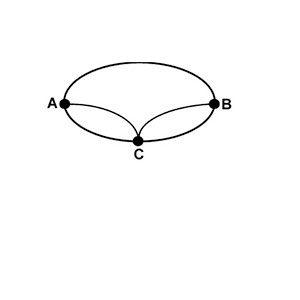### Question 2

I am collecting tokens and already have 12 and collect another 4 each week. When I have 100 I will be able to exchange them for a free meal. When will I be able to do this? A friend has 40 tokens and collects 3 each week. Which of us will be the first to get their free meal?

### Question 3

In the figure below AD = 4, AB = 3, and CD = 9. What is the area of triangle AEC? Note: As a reminder, the area of a triangle is ½ (base x height) and the diagram is not drawn to scale.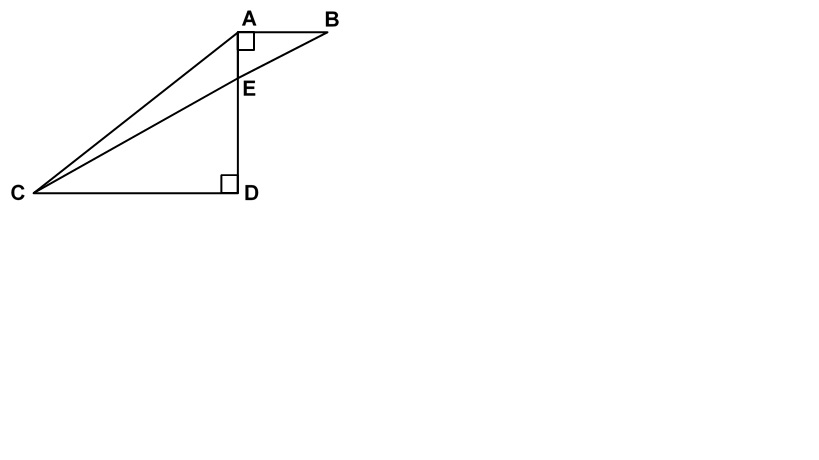### Question 4

a and b are positive real numbers with a > b which satisfy the equations
ab = 56/(a – b); a2 + b2 = 113/(a – b)
What is a + b?

### Question 1

Fiona leaves home at 8:30 am on Monday. She averages 60 mph from the time she leaves home until she stops for lunch at 12:00 am. She has travelled 3/5 of the way to her destination. After a 30-minute lunch break, she continues her journey at a slower average rate of 40 mph due to unfavourable weather conditions. What time does she reach her destination?

Solution

Miles travelled = 60 mph x 3.5 hrs. = 210 miles
1/5 distance travelled = 210 / 3 = 70 miles
2/5 distance remaining = 2 x 70 = 140 miles
Time for travelling remaining distance = 140 / 40 = 3.5 hours
Total time for driving & lunch = 3.5 + 0.5 + 3.5 = 7.5 hours
Arrival time = 7.5 hours after 8:30 am = 4 pm

### Question 2

How many triangles of any size are in the following diagram?

Solution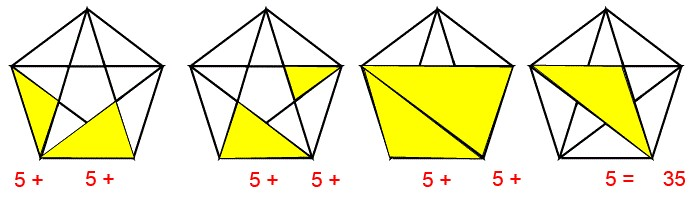The first three diagrams show pairs of shaded triangles and the fourth diagram shows a seventh triangle. The triangles exist in four additional positions (by rotating these diagrams) giving us 7 x 5 = 35 different triangles.

### Question 3

I have a box without a lid with dimensions 5 x 5 x 5. I place 125 cubes of
unit size in it. How many of these cubes have just one face touching the box?

Solution

There are 25 cubes touching each side of the box. Of this 25 the 5 cubes at the
bottom and the two sets of 4 cubes which neighbour the adjoining vertical
sides of the box have two faces touching the box. That leaves 12 cubes which
have just one face touching the box. So there are 4 x 12 = 48 cubes along the
vertical walls which have only one interface with the box.
At the bottom of the box the interior cubes are 3 x 3 = 9 in number
So overall there are 48 + 9 = 57 cubes with exactly one face touching the box.

### Question 4

In the right angled triangle below AM bisects the angle at A and meets the side BC at M with BM = 1 cm and MC = 2 cms. What is the length of AM?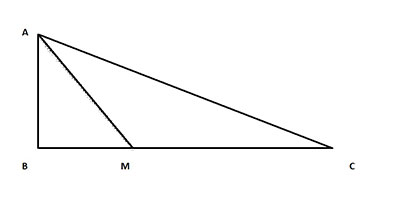Solution

Draw a line from M to meet AC at a right angle at K.
A B M C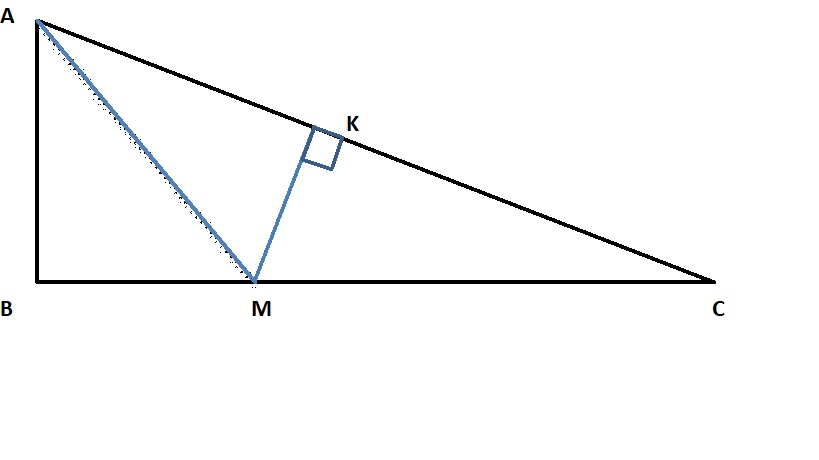As angles BAM and MAK are the same and ABM and MKA are right angles and AM is common to both triangles BAM and MAK, then those triangles are congruent.
Hence MK = BM = 1 so CK2 = CM2 – BM2 = 4 – 1 = 3 so CK = √3
AC2 = (AK + CK)2 = (AB + √3)2 = AB2 + 2√3AB + 3
AC2 = AB2 + BC2 = AB2+ 9
So 2√3AB = 6
AB = √3
AM2 = AB2 + BM2 = 3 + 1 so AM =2 cms.